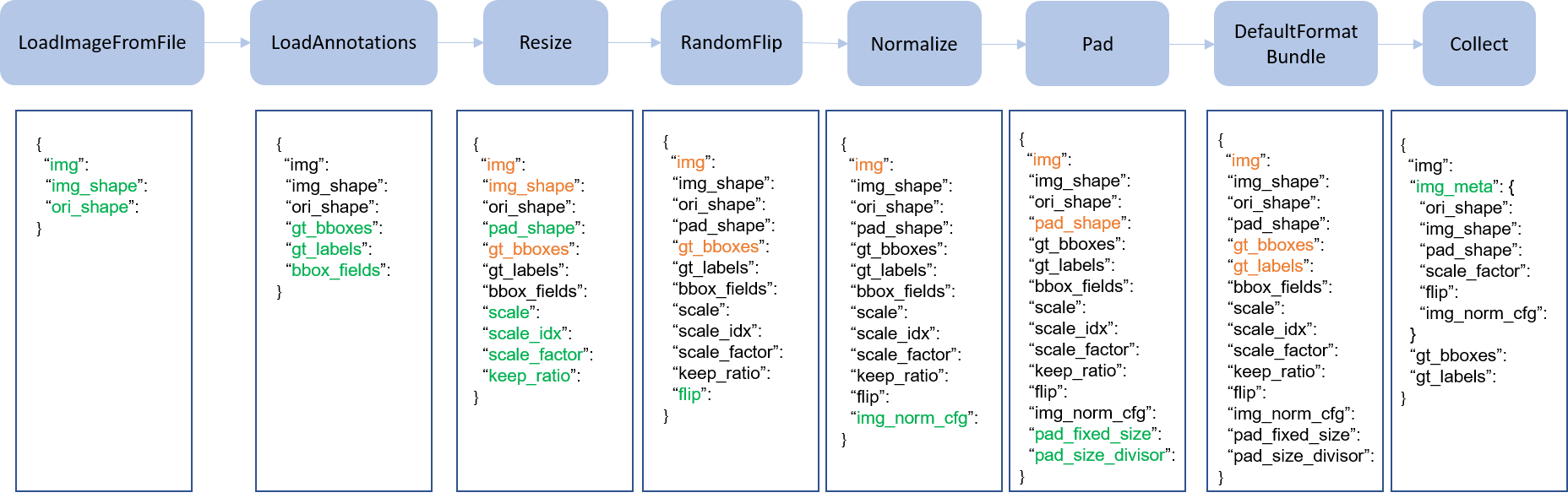# mmdet 3: Customize Data Pipelines

## Design of Data pipelines

Following typical conventions, we use `Dataset` and `DataLoader` for data loading with multiple workers. `Dataset` returns a dict of data items corresponding the arguments of models’ forward method. Since the data in object detection may not be the same size (image size, gt bbox size, etc.), we introduce a new `DataContainer` type in MMCV to help collect and distribute data of different size. See here for more details.

The data preparation pipeline and the dataset is decomposed. Usually a dataset defines how to process the annotations and a data pipeline defines all the steps to prepare a data dict. A pipeline consists of a sequence of operations. Each operation takes a dict as input and also output a dict for the next transform.

We present a classical pipeline in the following figure. The blue blocks are pipeline operations. With the pipeline going on, each operator can add new keys (marked as green) to the result dict or update the existing keys (marked as orange).The operations are categorized into data loading, pre-processing, formatting and test-time augmentation.

Here is a pipeline example for Faster R-CNN.

```img_norm_cfg = dict(
mean=[123.675, 116.28, 103.53], std=[58.395, 57.12, 57.375], to_rgb=True)
train_pipeline = [
dict(type='Resize', img_scale=(1333, 800), keep_ratio=True),
dict(type='RandomFlip', flip_ratio=0.5),
dict(type='Normalize', **img_norm_cfg),
dict(type='DefaultFormatBundle'),
dict(type='Collect', keys=['img', 'gt_bboxes', 'gt_labels']),
]
test_pipeline = [
dict(
type='MultiScaleFlipAug',
img_scale=(1333, 800),
flip=False,
transforms=[
dict(type='Resize', keep_ratio=True),
dict(type='RandomFlip'),
dict(type='Normalize', **img_norm_cfg),
dict(type='ImageToTensor', keys=['img']),
dict(type='Collect', keys=['img']),
])
]
```

For each operation, we list the related dict fields that are added/updated/removed.

`LoadImageFromFile`

`LoadAnnotations`

`LoadProposals`

### Pre-processing

`Resize`

• update: img, img_shape, *bbox_fields, *mask_fields, *seg_fields

`RandomFlip`

• update: img, *bbox_fields, *mask_fields, *seg_fields

`Pad`

`RandomCrop`

`Normalize`

• update: img

`SegRescale`

• update: gt_semantic_seg

`PhotoMetricDistortion`

• update: img

`Expand`

• update: img, gt_bboxes

`MinIoURandomCrop`

• update: img, gt_bboxes, gt_labels

`Corrupt`

• update: img

### Formatting

`ToTensor`

• update: specified by `keys`.

`ImageToTensor`

• update: specified by `keys`.

`Transpose`

• update: specified by `keys`.

`ToDataContainer`

• update: specified by `fields`.

`DefaultFormatBundle`

• update: img, proposals, gt_bboxes, gt_bboxes_ignore, gt_labels, gt_masks, gt_semantic_seg

`Collect`

• add: img_meta (the keys of img_meta is specified by `meta_keys`)
• remove: all other keys except for those specified by `keys`

### Test time augmentation

`MultiScaleFlipAug`

## Extend and use custom pipelines

1. Write a new pipeline in a file, e.g., in `my_pipeline.py`. It takes a dict as input and returns a dict.import random from mmdet.datasets import PIPELINES @PIPELINES.register_module() class MyTransform: “””Add your transform Args: p (float): Probability of shifts. Default 0.5. “”” def __init__(self, p=0.5): self.p = p def __call__(self, results): if random.random() > self.p: results[‘dummy’] = True return results
2. Import and use the pipeline in your config file. Make sure the import is relative to where your train script is located.custom_imports = dict(imports=[‘path.to.my_pipeline’], allow_failed_imports=False) img_norm_cfg = dict( mean=[123.675, 116.28, 103.53], std=[58.395, 57.12, 57.375], to_rgb=True) train_pipeline = [ dict(type=‘LoadImageFromFile’), dict(type=‘LoadAnnotations’, with_bbox=True), dict(type=‘Resize’, img_scale=(1333, 800), keep_ratio=True), dict(type=‘RandomFlip’, flip_ratio=0.5), dict(type=‘Normalize’, **img_norm_cfg), dict(type=‘Pad’, size_divisor=32), dict(type=‘MyTransform’, p=0.2), dict(type=‘DefaultFormatBundle’), dict(type=‘Collect’, keys=[‘img’, ‘gt_bboxes’, ‘gt_labels’]), ]
3. Visualize the output of your augmentation pipelineTo visualize the output of your augmentation pipeline, `tools/misc/browse_dataset.py` can help the user to browse a detection dataset (both images and bounding box annotations) visually, or save the image to a designated directory. More detials can refer to useful_tools
Categories: vision

#### onesixx

Blog Owner